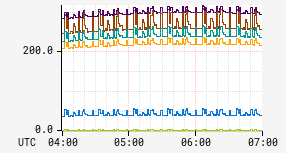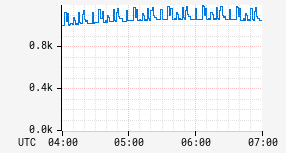# sum

Sum aggregation operator. There are two variants of the `:sum` operator.

## Aggregation¶

Input Stack:
 Query
Output Stack:
 AggregationFunction

Compute the sum of all the time series that match the query. Sum is the default aggregate used if a query is specified with no explicit aggregate function. Example with implicit sum:

```name,ssCpuUser,:eq
```

Equivalent example with explicit sum:

```name,ssCpuUser,:eq,
:sum
```

When matching against the sample data in the table below, the highlighted time series would be included in the aggregate result:

Namenf.appnf.nodeData
ssCpuUser alerttest i-0123 [1.0, 2.0, NaN]
ssCpuSystem alerttest i-0123 [3.0, 4.0, 5.0]
ssCpuUser nccp i-0abc [8.0, 7.0, 6.0]
ssCpuSystem nccp i-0abc [6.0, 7.0, 8.0]
numRequests nccp i-0abc [1.0, 2.0, 4.0]
ssCpuUser api i-0456 [1.0, 2.0, 2.0]

The values from the corresponding intervals will be aggregated. For the first interval using the sample data above the values are `1.0`, `8.0`, and `1.0`. Each value other than `NaN` contributes one to the sum. This leads to a final result of:

NameData
ssCpuUser [10.0, 11.0, 8.0]

The only tags for the aggregated result are those that are matched exactly (:eq clause) as part of the choosing criteria or are included in a group by.

## Math¶

Input Stack:
 TimeSeriesExpr
Output Stack:
 TimeSeriesExpr

Compute the sum of all the time series from the input expression. This is typically used when there is a need to use some other aggregation for the grouping. Example:

 Before After```name,sps,:eq, :max, (,nf.cluster,),:by ``` ```name,sps,:eq, :max, (,nf.cluster,),:by, :sum ```